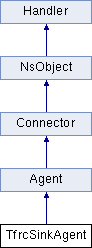# TfrcSinkAgent Class Reference

Inheritance diagram for TfrcSinkAgent:List of all members.

## Public Member Functions

void recv (Packet *, Handler *)

## Protected Member Functions

void sendpkt (double)
void nextpkt (double)
double adjust_history (double)
double est_loss ()
double est_thput ()
int command (int argc, const char *const *argv)
void print_loss (int sample, double ave_interval)
void print_loss_all (int *sample)
void print_losses_all (int *losses)
void print_count_losses_all (int *count_losses)
void print_num_rtts_all (int *num_rtts)
int new_loss (int i, double tstamp)
double estimate_tstamp (int before, int after, int i)
double est_loss_WALI ()
void shift_array (int *a, int sz, int defval)
void shift_array (double *a, int sz, double defval)
void multiply_array (double *a, int sz, double multiplier)
void init_WALI ()
double weighted_average (int start, int end, double factor, double *m, double *w, int *sample)
int get_sample (int oldSample, int numLosses)
int get_sample_rtts (int oldSample, int numLosses, int rtts)
double weighted_average1 (int start, int end, double factor, double *m, double *w, int *sample, int ShortIntervals, int *losses, int *count_losses, int *num_rtts)
double est_loss_EWMA ()
double est_loss_RBPH ()
double est_loss_EBPH ()

## Protected Attributes

TfrcNackTimer nack_timer_
int psize_
int fsize_
double rtt_
double tzero_
int smooth_
int total_received_
int total_losses_
int total_dropped_
int bval_
double last_report_sent
double NumFeedback_
int rcvd_since_last_report
int losses_since_last_report
int printLoss_
int maxseq
int maxseqList
int numPkts_
double minDiscountRatio_
int numPktsSoFar_
int PreciseLoss_
int ShortIntervals_
int ShortRtts_
double last_timestamp_
double last_arrival_
int hsz
char * lossvec_
double * rtvec_
double * tsvec_
int lastloss_round_id
int round_id
double lastloss
int numsamples
int * sample
double * weights
double * mult
int * losses
int * count_losses
int * num_rtts
double mult_factor_
int sample_count
int last_sample
int init_WALI_flag
int loss_seen_yet
int adjust_history_after_ss
int false_sample
int algo
int discount
int bytes_
double history
double avg_loss_int
int loss_int
double sendrate
int minlc

## Friends

class TfrcNackTimer

## Member Function Documentation

 double TfrcSinkAgent::est_loss_WALI ( ) ` [protected]`

WALI Code

The documentation for this class was generated from the following files:
• /Users/yzchen/ns/ns-allinone-2.33/ns-2.33/tcp/tfrc-sink.h
• /Users/yzchen/ns/ns-allinone-2.33/ns-2.33/tcp/tfrc-sink.cc